# WEEKDAY Function

Returns an integer that represents the day of the week for a given date

## What is the WEEKDAY Function?

The WEEKDAY Function is an Excel DATE and TIME Function. The function will return an integer that is a representation of the day of the week for a given date.

WEEKDAY is quite useful in financial analysis. Suppose we wish to determine the time required to complete a certain project – the function can be useful in removing weekends from the given time frame. Thus, it is particularly useful when planning and scheduling work for a business project.

### Formula

=WEEKDAY(serial_number,[return_type])

It uses the following arguments:

1. Serial_number (required argument) – This is a sequential number representing the date of the day that we are trying to find.
2. Return_type (optional argument) – This specifies which integers are to be assigned to each weekday. Possible values are:

Return_typeNumber returned
1 or omittedIt will take Sunday as first day of week so, Sunday = 1, Monday = 2, ... , Saturday = 7
2It will take Monday as first day of week so, Monday = 1, Tuesday = 2, ... , Sunday = 7
3It will take Tuesday as first day of week so, Monday = 0, Tuesday = 1, ... , Sunday = 6
11Monday = 1, Tuesday = 2, ... , Sunday = 7
12Tuesday = 1, Wednesday = 2, ... , Monday = 7
13It will take Wednesday as first day of week so, Wednesday = 1, Thursday = 2, ... , Tuesday = 7
14It will take Thursday as first day of week so, Thursday = 1, Friday = 2, ... , Wednesday = 7
15It will take Friday as first day of week so, Friday = 1, Saturday = 2, ... , Thursday = 7
16It willl take Saturday as first day of week so, Saturday = 1, Sunday = 2, ... , Friday = 7
17Sunday = 1, Monday = 2, ... , Saturday = 7

### A few notes about the WEEKDAY Function:

1. If we omit the return_type argument, it will take the default value of 1.
2. Microsoft Excel stores dates as sequential serial numbers so they can be used in calculations. By default, January 1, 1900 is serial number 1, and January 1, 2009 is serial number 39448 because it is 39,813 days after January 1, 1900. Excel interprets text representations of dates differently, depending on the date settings on your computer. Therefore, the serial_number argument for the WEEKDAY function should be entered as either:
1. A reference to a cell containing a date
2. A date returned from another function or formula.

### How to use the WEEKDAY Function in Excel?

It is a built-in function that can be used as a worksheet function in Excel. To understand the uses of WEEKDAY, let us consider a few examples:

#### Example 1

Let’s see how we can get weekends and weekdays highlighted. It can be done using conditional formatting rules. Using the data below: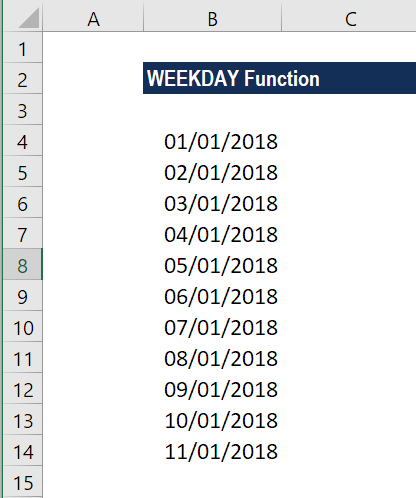Using the WEEKDAY formula, we can find out if the day is a weekday or weekend. The formula to use will be: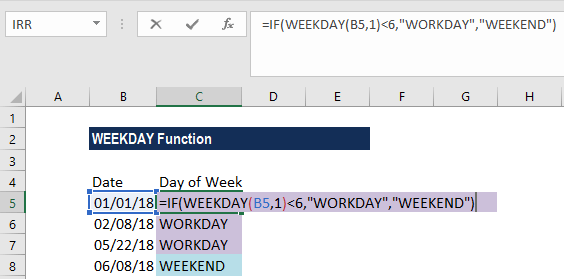We get the results below: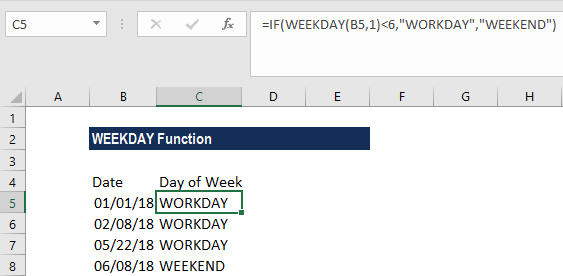Here, we’ve taken the return_type as 1 as we want Sunday to be the first day of the week. So, here a Friday would be a weekend as per this formula.

Now to improve the presentation of the data, we can use conditional formatting to highlight weekdays, which is the working days and weekends, in different colors.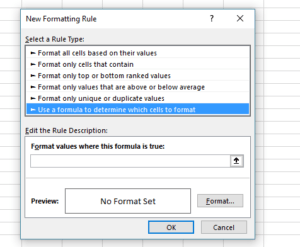We will create two rules in conditional formatting where the first rule would be WEEKDAY(\$A2,1)<6 and second rule would be =WEEKDAY(\$A2,1)>5.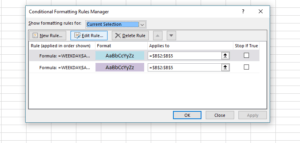We get the results below: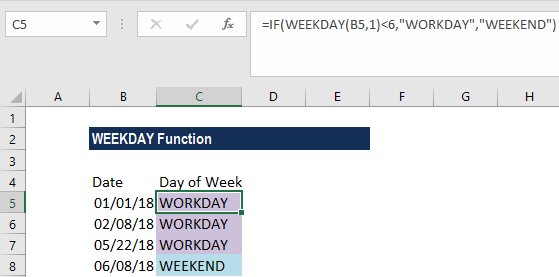#### Example 2

If we wish to get the day names in any format of our choice, we can use the WEEKDAY function along with the CHOOSE function. Using the data below: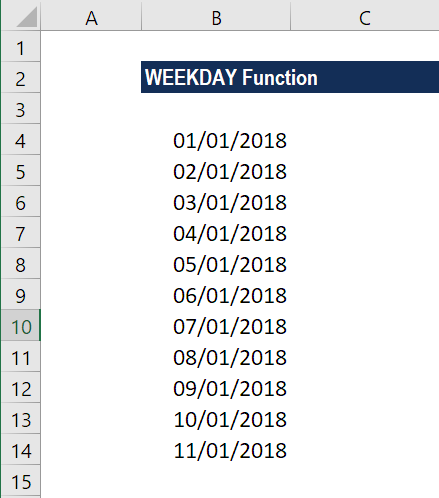Now we want the day to be displayed as Sun, Mon, Tue, Wed, Thur, Fri and Sat. The formula to use will be: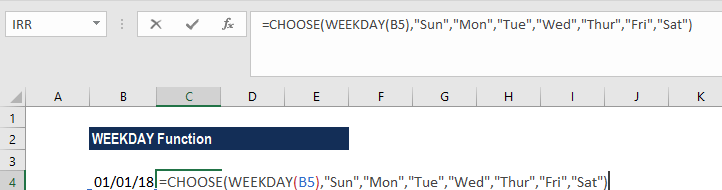In the formula above, the WEEKDAY function will get the day of week as a serial number. Then, the CHOOSE function will use that number as index_num (the first argument) that indicates which value from the list of value arguments to return. The results we get are shown below: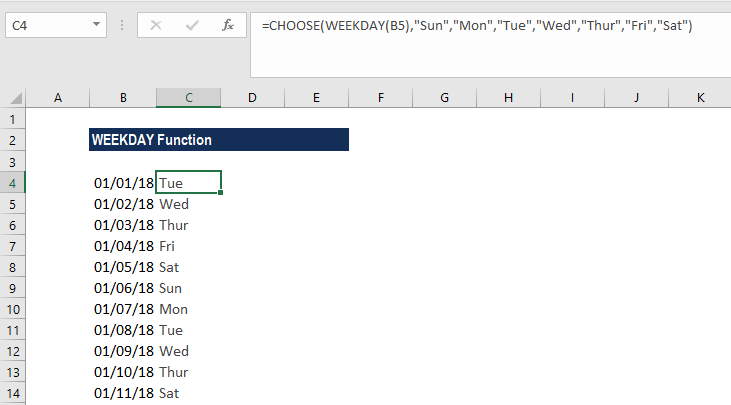### Common errors in the WEEKDAY Function:

1. #VALUE! error – Occurs when either the given serial_number or the given [return_type] is non-numeric.
2. #NUM! error – Occurs when:
• The given serial_number argument is numeric but is out of range for the current date base.
• The given return_type argument is not one of the permitted values (1-3 or 11-17).

Thanks for reading CFI’s guide to important Excel functions! By taking the time to learn and master these functions, you’ll significantly speed up your financial modeling. To learn more, check out these additional CFI resources: